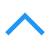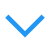# 深度学习基础网络 ResNet

## Highway Networks

$y = H(x,W_H)\tag 1$

highway network添加了两个非线性转换: transform gate $T(x,W_T)$ ,carry gate $C(x,W_C)$:

$y = H(x,W_H)\cdot T(x,W_T) +x\cdot C(x,W_C)\tag 2$

$C = 1 − T$,得到

$y = H(x,W_H)\cdot T(x,W_T) +x\cdot (1-T(x,W_T))\tag 3$

$T(x,W_T)=0$时,$y=x$;当$T(x,W_T)=1$时,$y=H(x,W_H)$.因此这个gate可以灵活地控制网络的行为.直观的理解就是每层不完全做非线性特征变换了,将原始特征直接添加到这一层,更有弹性一些.

Highway network学习之后自动学习哪些层需要哪些不需要.而ResNet是直接(等比例)相加.

## ResNet

ResNet与Highway Networks的区别是Highway Networks增加了额外的参数,而ResNet不需要;Highway Networks,当carry gate接近于0时,残差功能接近关闭状态.而ResNet的残差函数总是开启.### Identity mapping（恒等映射）Identity mapping反向求导时正好是单位矩阵I.

$\mathbb y=\mathcal F(\mathbb x,{W_i})+\mathbb x \tag 1$

$\mathbb y=\mathcal F(\mathbb x,{W_i})+W_s\mathbb x \tag 2$

### Plain Network

Plain Network 主要是受 VGG 网络启发，主要采用3x3滤波器，遵循两个设计原则：

1. 输出相同特征图尺寸的卷积层，有相同个数的滤波器(stride=1使得输出尺寸不变)
2. 通过stride=2降采样后特征图尺寸缩小一半，增加一倍的滤波器个数使每层卷积的计算复杂度相同(以特征图大小56->28为例,56的卷积操作数为(56x56x64)x(3x3x64),而64的卷积操作数为(28x28x128)x(3x3x128),计算量相等,当然stride=2的conv层计算量减半).

### Residual Network

A. 使用恒等映射，如果residual block的输入输出维度不一致，对增加的维度用0来填充；

B. 在block输入输出维度一致时使用恒等映射，不一致时使用线性投影以保证维度一致(使用一层Conv+BatchNorm即可)；

C. 对于所有的block均使用线性投影。

Deeper Bottleneck结构:Plain Network,Residual Network与VGG-19的区别:### ResNet解读ResNet只是表面上看起来很深，事实上网络却很浅。

### 代码实现

caffe中实现特征的加法,用Eltwise层的SUM operation即可:

layer {
name: "Eltwise3"
type: "Eltwise"
bottom: "Eltwise2"
bottom: "Convolution7"
top: "Eltwise3"
eltwise_param {
operation: SUM
}
}

posted @ 2018-03-22 09:25  康行天下  阅读(9824)  评论(4编辑  收藏  举报